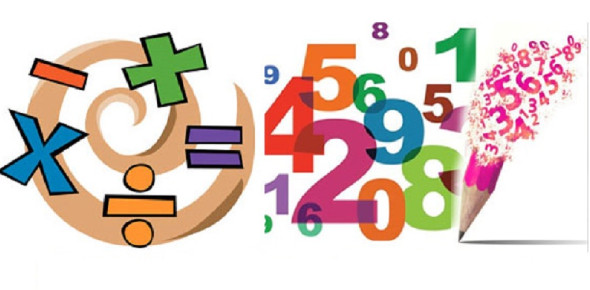# Cat Mock Test: Quantitative Aptitude

20 Questions | Attempts: 2874Settings.

• 1.
The sum of n terms of the series 2.2 + 5.02 + 8.002 + 11.0002 + …. is
• A.
• B.
• C.
• D.
• 2.
• A.
• B.
• C.
• D.
• 3.
Number of students who have opted for the subjects A, B and C are 60, 84 and 108 respectively. The examination is to be conducted for these students such that only the students of the same subject are allowed in one room. Also, the number of students in each room must be same. What is the minimum number of rooms that should be arrange to meet all these conditions?
• A.

28

• B.

60

• C.

12

• D.

21

• 4.
In how many ways can be number 44100 can be written as a product of two different factors?
• A.

30

• B.

60

• C.

50

• D.

40

• 5.
The base of the rectangular solid is a square, and its height is twice its length. If its volume is 16000m2,
• A.

4200 m2

• B.

4800 m2

• C.

4000 m2

• D.

4500 m2

• 6.
The sum of interior angles of a polygon is 1440o. How many sides are there in the polygon?
• A.

8

• B.

10

• C.

11

• D.

12

• 7.
The base of a prism is a trapezium whose parallel sides are 25m and 13m respectively, the distance between them being 6m. Find the volume of the prism if its height is 22m.
• A.

2518m3

• B.

2516m3

• C.

2508m3

• D.

2808m3

• 8.
A line passes though the point (3, 4) and cuts off intercepts from the co-ordinates axes such that their sum is 14. The equation of the line is:
• A.
• B.
• C.
• D.
• 9.
• A.
• B.
• C.
• D.
• 10.
Sum of the radius of the base and the height of a solid cylinder is 10cm. If the total surface of the cylinder is 80 π sq. cm, the volume of the cylinder is.
• A.
• B.
• C.
• D.
• 11.
A toy has a hemisphere base and a conical top as shown in the figure. The perpendicular height of the cone is 10 cm and radius of the hemisphere is 4 cm. Find the volume of the toy.
• A.

302cu. Cm.

• B.

320cu. Cm.

• C.

203cu. Cm.

• D.

230cu. Cm.

• 12.
Three pieces of cakes of weights  lbs,  lbs, and  lbs respectively are to be divided into parts of equal weights. Further, each part must be as heavy as possible. If one such part is served to each guest, then what is the maximum number of guests that could be entertained?
• A.

54

• B.

72

• C.

20

• D.

21

• 13.
What is the number of distinct triangles with integral valued sides and perimeter as 14?
• A.

6

• B.

5

• C.

4

• D.

3

• 14.
The area of the three faces of a cuboid are in the ratio 1:3:4 and its volume is 144 c.c. The length of its diagonal is
• A.

17 cm

• B.

21 cm

• C.

13 cm

• D.

24 cm

• 15.
If (9, a) is at the distance of 5 units from the point (a, 2), find 'a'.
• A.

5

• B.

6

• C.

5 or 6

• D.

Neither 5 nor 6

• 16.
If p is a prime number greater than  is not divisible by which of the following?
• A.

6

• B.

24

• C.

2

• D.

None of these

• 17.
The radii of two cylinders are in the ratio 2 : 2; and the heights are in the ratio 5 : 3; calculate the ratio of their volumes.
• A.
• B.
• C.
• D.

None of these

• 18.
What is the sum of the first 100 three digit numbers?
• A.

14950

• B.

15000

• C.

15050

• D.

1600

• 19.
Find the distance between the parallel lines 3x + 4y - 10 = 0 and 6x + 8y + 15 = 0
• A.

3.4 Units

• B.

5.3 Units

• C.

3.2 Units

• D.

2.5 Units

• 20.
A 4cm cube is cut into 1cm cues. What is the percentage increase in the surface area after such cutting?
• A.

4%

• B.

300%

• C.

75%

• D.

400%

## Related TopicsBack to top
×

Wait!
Here's an interesting quiz for you.